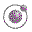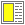Name binding, Recursion, Iteration, and Continuations - slide 36 : 42

The intuition behind continuations

A continuation of the evaluation of an expression E in a surrounding context C represents the future of the computation, which waits for, and depends on, the value of E

 Context C and expression E Intuitive continuation of E in C `(+ 5 (* 4 3))` The adding of 5 to the value of E `(cons 1 (cons 2 (cons 3 '())))` The consing of 3, 2 and 1 to the value of E ```(define x 5) (if (= 0 x) 'undefined (remainder (* (+ x 1) (- x 1)) x))``` The multiplication of E by x - 1 followed by a division by x